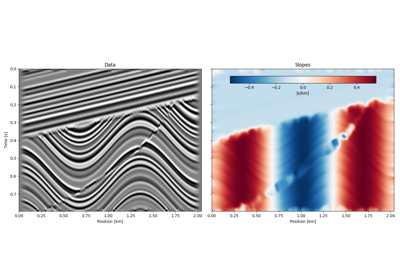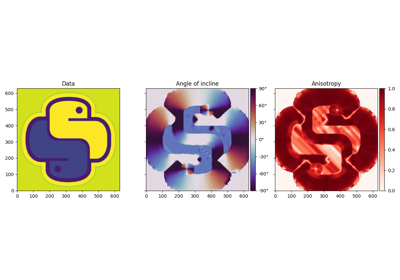# pylops.utils.signalprocessing.slope_estimate#

pylops.utils.signalprocessing.slope_estimate(d, dz=1.0, dx=1.0, smooth=5, eps=0.0, dips=False)[source]#

Local slope estimation

Local slopes are estimated using the Structure Tensor algorithm . Slopes are returned as $$\tan\theta$$, defined in a RHS coordinate system with $$z$$-axis pointing upward.

Note

For stability purposes, it is important to ensure that the orders of magnitude of the samplings are similar.

Parameters
dnp.ndarray

Input dataset of size $$n_z \times n_x$$

dzfloat, optional

Sampling in $$z$$-axis, $$\Delta z$$

Warning

Since version 1.17.0, defaults to 1.0.

dxfloat, optional

Sampling in $$x$$-axis, $$\Delta x$$

Warning

Since version 1.17.0, defaults to 1.0.

smoothfloat or np.ndarray, optional

Standard deviation for Gaussian kernel. The standard deviations of the Gaussian filter are given for each axis as a sequence, or as a single number, in which case it is equal for all axes.

Warning

Default changed in version 1.17.0 to 5 from previous value of 20.

epsfloat, optional

New in version 1.17.0.

Regularization term. All slopes where $$|g_{zx}| < \epsilon \max_{(x, z)} \{|g_{zx}|, |g_{zz}|, |g_{xx}|\}$$ are set to zero. All anisotropies where $$\lambda_\text{max} < \epsilon$$ are also set to zero. See Notes. When using with small values of smooth, start from a very small number (e.g. 1e-10) and start increasing by a power of 10 until results are satisfactory.

dipsbool, optional

New in version 2.0.0.

Return dips (True) instead of slopes (False).

Returns
slopesnp.ndarray

Estimated local slopes. The unit is that of $$\Delta z/\Delta x$$.

Warning

Prior to version 1.17.0, always returned dips.

anisotropiesnp.ndarray

Estimated local anisotropies: $$1-\lambda_\text{min}/\lambda_\text{max}$$

Note

Since 1.17.0, changed name from linearity to anisotropies. Definition remains the same.

Notes

For each pixel of the input dataset $$\mathbf{d}$$ the local gradients $$g_z = \frac{\partial \mathbf{d}}{\partial z}$$ and $$g_x = \frac{\partial \mathbf{d}}{\partial x}$$ are computed and used to define the following three quantities:

\begin{split}\begin{align} g_{zz} &= \left(\frac{\partial \mathbf{d}}{\partial z}\right)^2\\ g_{xx} &= \left(\frac{\partial \mathbf{d}}{\partial x}\right)^2\\ g_{zx} &= \frac{\partial \mathbf{d}}{\partial z}\cdot\frac{\partial \mathbf{d}}{\partial x} \end{align}\end{split}

They are then spatially smoothed and at each pixel their smoothed versions are arranged in a $$2 \times 2$$ matrix called the smoothed gradient-square tensor:

$\begin{split}\mathbf{G} = \begin{bmatrix} g_{zz} & g_{zx} \\ g_{zx} & g_{xx} \end{bmatrix}\end{split}$

Local slopes can be expressed as $$p = \frac{\lambda_\text{max} - g_{zz}}{g_{zx}}$$, where $$\lambda_\text{max}$$ is the largest eigenvalue of $$\mathbf{G}$$.

Similarly, local dips can be expressed as $$\tan(2\theta) = 2g_{zx} / (g_{zz} - g_{xx})$$.

Moreover, we can obtain a measure of local anisotropy, defined as

$a = 1-\lambda_\text{min}/\lambda_\text{max}$

where $$\lambda_\text{min}$$ is the smallest eigenvalue of $$\mathbf{G}$$. A value of $$a = 0$$ indicates perfect isotropy whereas $$a = 1$$ indicates perfect anisotropy.

1

Van Vliet, L. J., Verbeek, P. W., “Estimators for orientation and anisotropy in digitized images”, Journal ASCI Imaging Workshop. 1995.

## Examples using pylops.utils.signalprocessing.slope_estimate#Seislet transform

Seislet transformSlope estimation via Structure Tensor algorithm

Slope estimation via Structure Tensor algorithm